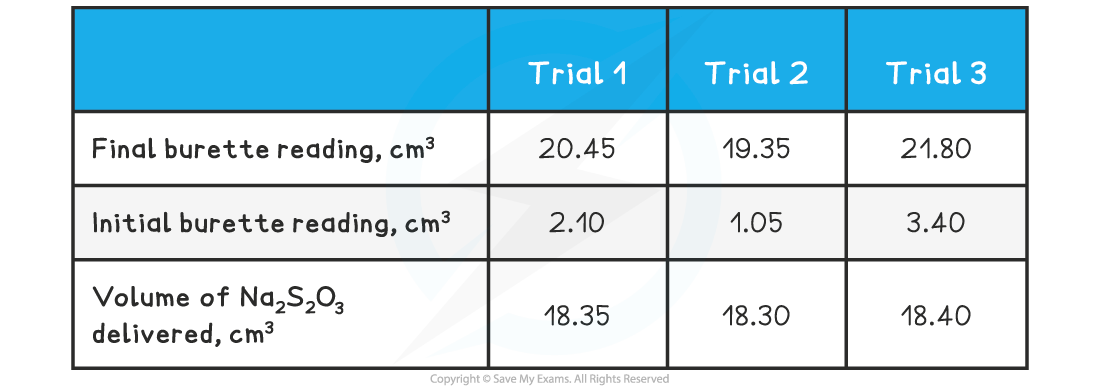# IB DP Chemistry: SL复习笔记9.1.8 The Winkler Method

### The Winkler Method

• The Winkler Method is a technique used to measure dissolved oxygen in freshwater systems
• Dissolved oxygen is used as an indicator of the health of a water body, where higher dissolved oxygen concentrations correlate with high productivity and little pollution
•  The biological oxygen demand (BOD) is the amount of oxygen used to decompose the organic matter in a sample of water over a specified time period, usually 5 days, at a specified temperature
• high BOD indicates a greater quantity of organic waste in the water, which means a lower level of dissolved oxygen

#### Chemical analysis

• In the first step, manganese(II) sulfate is added to a water sample and then the solution is made alkaline with NaOH
• In the alkaline solution, dissolved oxygen will oxidize manganese(II) ions to manganese(IV)

2Mn2+(aq)  +   4OH(aq) + O(aq) → 2MnO(s)   + 2H2O (l)

• The manganese(IV) oxide, MnO2, appears as a brown precipitate
• In the second part, acidified potassium iodide, KI, is added to the solution
• The precipitate will dissolve back into solution, so Mn(IV) is reduced back to Mn(II) liberating iodine in the process

MnO2 (s)  +  2I(aq) + 4H+ (aq)  → Mn2+(aq) + I2(aq) + 2H2O(l)

• Thiosulfate is used, with a starch indicator, to titrate the iodine liberated

2S2O32−(aq) + I2 (aq) → S4O62−(aq) + 2I(aq)

• From the above stoichiometric equations, we can see that:

1 mole of O2 → 2 moles of MnO→ 2 moles of I→ 4 moles of S2O32−

• Therefore, after determining the number of moles of iodine produced, we can work out the number of moles of oxygen molecules present in the original water sample
• The oxygen content is usually presented as mg/dmor ppm

### Winkler Method Calculation

• The following calculation shows how the data from a Winkler analysis is carried out:

#### Worked Example

A sample of lake water was analysed using the Winkler Method. The size of the sample was 600 cm3 and the following table shows the results of a titration of the liberated iodine against 0.0500 mol dm-3 sodium thiosulfate solution in the final step of the analysis:Determine the dissolved oxygen content of the water sample in g dm-3

Step 1: Determine the average volume of  delivered

Average volume Na2S2O =  (18.35 + 18.30 + 18.40) ÷ 3

Average volume Na2S2O = 18.35 cm3

Step 2: Determine the number of moles of sodium thiosulfate reacted

Moles of Na2S2O= volume in dm3 x concentration = 0.01835 dm3 x 0.0500 mol dm-3= 9.175 x 10-4 mol

Step 3: Determine the moles of oxygen reacted

1 mol of O2 is equivalent to 4 mol of Na2S2O3

Therefore, amount of oxygen in the sample is = (9.175 x 10-4) ÷ 4  = 2.294 x 10-4  mol

Step 4: Calculate the concentration of the dissolved oxygen

Concentration = mol ÷ volume in dm3 = (2.294 x 10-4  mol) ÷ 0.600 dm3= 3.823 x 10-4  mol dm-3

Convert to g dm-3 = 3.823 x 10-4 mol dm-3  x 32.00 g mol-1 = 0.01223 g dm-3